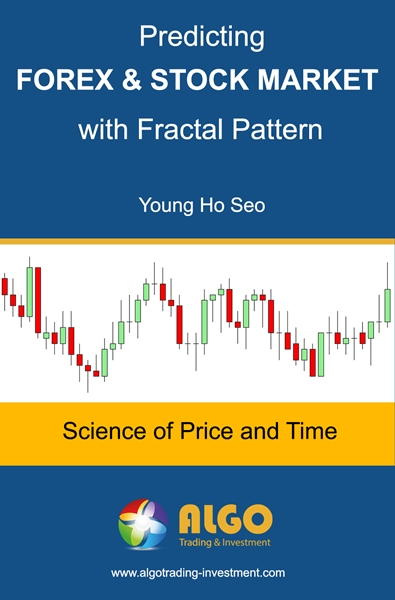•### Predicting Forex and Stock Market with Fractal Pattern

Subtitle: Science of Price and Time

Author: Young Ho Seo

Financial Trader, Engineer, and Quantitative Developer

Publication Date: 11 April 2020

Total Pages counted in MS-Word: 330

Total Words counted in MS-Word: 39,000

List of our E-Book Distributor

Just click link below to purchase our book from other Reputable Distributors. Use one of this reliable distributor to buy our eBooks.

Also you can visit this link to view all the distributors of our EBook:

This book provides you the powerful and brand new knowledge on predicting financial market that we have discovered in several years of own research and development work. This book will help you to turn your intuition into the scientific prediction method. In the course of recognizing many price patterns in the chart of Forex and Stock market, you should be realized that it was your intuition working at the background for you. The geometric prediction equation devised in this book will provide you the scientific way to predict the financial market. It is simple and easy. What is even better is that this approach is aligned with your intuition.
Many of us had a mistake of viewing the financial market with deterministic cycle. Even though we knew that market would not land us such a simple prediction pattern, we never stop using the concept of deterministic cycle to predict them, for example, using Fourier transform. Why is that so? The reason is simply that no one presented an effective way of predicting stochastic cycle. Stochastic cycle is the true face of the financial market when many variables in the market are suppressing the predictable cycle with fixed time interval. So how we predict the stochastic cycle present in the financial market? We present the answer in this book. We show that the geometric prediction equation solves the stochastic cycle modelling problem together with fractal wave in the financial market. In another words, your intuition, more precisely your capability to recognize geometric shape, is more powerful than any other technical indicators available in the market. Hence, the geometric prediction would maximize your ability to predict the financial market.
Geometric prediction combines your ability to recognize the geometric regularity and statistical regularity from the chart in Forex and Stock market data. We provide how the powerful chart patterns are related to geometric regularity through your intuition. The chart patterns covered in this book include support, resistance, Fibonacci Price pattern, Harmonic Pattern, Falling Wedge pattern, Rising Wedge pattern, and Gann Angles with probability. At the same time, we provide you how the turning point probability is related to statistical regularity. We show how the fractal wave can be understood using the relationship between mother waves and child waves. In this book, we demonstrate how to combine chart patterns with turning point probability to predict the financial market.

1 Introduction to Fractal Pattern in Financial Market
1.1 Geometric Recognition and Prediction, the Bible for Successful Trading
1.2 Turning Point, Peak, Trough, Swing High, Swing Low, and ZigZag
1.3 Fractal Wave, Mother Wave, and Child Wave
2 Geometric Regularity with Ratio and Proportion in Price Pattern
2.1 Finding Geometric Regularity within Fractal Waves
2.2 Detecting Price Patterns using Intersection
2.3 Detecting Price Patterns using Proportion
2.4 Detecting Price Patterns using Ratio
2.5 Application and Limitation of Price Patterns in Trading
3 Statistical Regularity with Size in Price Pattern
3.1 Time Series Decomposition and Fractal Decomposition Analysis (FDA)
3.2 Fourier Transform and Peak Trough Transform
3.3 Deterministic Cycle and Stochastic Cycle in Financial Market
3.4 Singular Spectrum Analysis (SSA) and Fractal Cycle Analysis (FCA)
3.5 Turning Point Probability in Price and Time
4 Trading with Stochastic Cycles using Fractal Wave
4.1 Fibonacci Probability Graph
4.2 Mother Wave and Child Wave with Joint Probability
4.3 Predicting Volatility with Turning Point Probability
4.4 Support and Resistance Trading with Turning Point Probability
4.5 Harmonic Pattern Trading with Turning Point Probability
4.6 Falling Wedge Pattern and Rising Wedge Pattern with Turning Point Probability
4.7 Gann Angles with Probability
4.8 Trading with Fractal Wave and Stochastic Cycles
5 Risk Management in the Financial Trading
5.1 Position Sizing Techniques
5.3 Breakeven Success (or win) Rate
5.5 Compounding Profits
6 Price Patterns in the Financial Market
6.1 Why Do We Need New Pattern Framework for day trading?
6.2 How the X3 Pattern Framework is different from other Approaches
6.3 Defining Price Patterns with Retracement and Expansion Ratio
6.4 Scientific Lag Notation for Retracement Ratio and Expansion Ratio
6.5 Closing Retracement Ratio to Describe the Structure of Pattern
6.6 Factored Expansion Ratio to Describe Structure of Pattern
6.7 Converting Number of Points to Number of Triangles
7 Pattern Notation with Name, Structure, and Ideal Ratios
7.1 Pattern with 1 Triangle (3 points) Examples
7.2 Pattern with 2 Triangles (4 points) Examples
7.3 Pattern with 3 Triangles (5 points) Examples
7.4 Pattern with 4 Triangles (6 points) Examples
7.5 Pattern with 7 Triangles (9 points) Examples
8 Tutorial with Peak Trough Analysis
8.2 Working with Fibonacci Retracement in Your Chart
8.3 Working with Fibonacci Expansion in Your Chart
9 Tutorial with X3 Price Pattern in Excel Spreadsheet
9.1 Calculating the Fibonacci Retracement Ratio in Microsoft
9.2 Calculating the Fibonacci Expansion Ratio in Apple
9.3 Detecting AB=CD pattern in Facebook
10 Special Chapter: Algorithm and Prediction for Artificial Intelligence, Time Series Forecasting, and Technical Analysis
11 ReferencesSubscribe
Notify of